# Improvement of Automatic Generation Control Behavior of Two Interconnected Areas Using SMES and TCSC

Improvement of Automatic Generation Control Behavior of Two Interconnected Areas Using SMES and TCSC
LACoSERE Laboratory University of Amar TELIDJI, Laghouat, Algeria

Corresponding Author Email:
bo.yousfi@lagh-univ.dz
Page:
40-45
|
DOI:
https://doi.org/10.18280/ama_b.641-406
9 March 2021
|
Accepted:
18 July 2021
|
Published:
31 December 2021
| Citation

OPEN ACCESS

Abstract:

In this paper, an attempt has been made to enhance the dynamic behaviour of Automatic Generation Control (AGC) of two areas two units using both Thyristor Controlled Series Compensator (TCSC) placed in the tie-line and Superconducting Magnetic Energy Storage (SMES) units are considered in both areas. For more realistic study, the effects of Governor Dead Band (GDB) and Generation Rate Constraints (GRCs) are taken into account for both areas. However, to conduct the system to better dynamic responses, we have implemented a PI-PD cascade controller. After that, a well-known and powerful optimisation algorithm named Firefly Algorithm (FA) is employed by evaluating the Integral of the Squared Error (ISE). From the obtained results, the implemented methods prove its efficiency from different view of points such as: minimisation of Overshoot and Undershoot Peaks (PO), (PU) and Settling Time (ST).

Keywords:

interconnected power system, two areas, non-linear constraints, PI-PD cascade, FACTS, FA, ISE

1. Introduction

At any moment, a power system operating condition should be stable, meeting various operational criteria, and it should also be protected in the presence of any emergency. Power system stability defined as the ability of the system to regain an acceptable state of equilibrium after being subjected to disturbances .

Nowadays, power systems have been operated closer to their stability limits due to economic and environmental constraints. Therefore, a stable and secure operation conditions present a very important challenge for the operators. Power system stability is mainly classified into three main categories as follows: rotor angle stability, frequency stability and voltage stability .

Frequency stability is defined as the ability of a power system to maintain a steady frequency within an acceptable range following a severe system disturbance resulting in a significant imbalance between generation and load .

For this reason, the Automatic Generation Control (AGC) has been introduced whose role is:

• to regulate the frequency to its specified nominal value;

• to maintain the interchange power between control areas at the scheduled values;

• to distribute the required change in generation among units to minimize the operating costs.

To attain these principal objectives, a linear equation called Area Control Error (ACE) has been used, which is associated with two main variables named as frequency deviation and tie-line power exchange. The ACE signal must be sets to zero in the case of any load variation in the system.

The AGC has been introduced with its four main actions (four control loops) as follows:

Primary Control Loop

Its main role is to re-establish a balance between generation and demand at frequency different from its nominal value.

Secondary Control Loop

This control also known as a Load Frequency Control (LFC) that allows to correcting the total power deviation.

Tertiary Control Loop

Typically, the operation of TCL which is the Economic Dispatch (ED), as a complementary to Secondary Control, is bound to the period of Scheduling but has in principle the same impact on the interconnected operation as SCL. The TCL is any automatic or manual change in the working points of generators (mainly by re-scheduling), to restore adequate Secondary Control Reserve (SCR) at the right time.

The Load Scheduling (LS) is the disconnection of loads from the synchronous electric system, usually performed automatically, to control the system frequency in emergency conditions.

The LFC problem in power systems has a long history. In a power system, LFC as an ancillary service acquires an important and fundamental role to maintain the electrical system reliability at an adequate level . The LFC scheme has evolved over the past few decades and is used on interconnected power systems. There has been continuing interest in designing LFC with better performance to maintain the frequency and keep tie-line power flows within prespecified values using various control strategies .

For more realistic study, several non-linear constraints such as Governor Dead Band (GDB), Generation Rate constraint (GRC), Dynamic Boiler (DB), Time Delay (TD) have been introduced [5-8].

In control system design and analysis, certain design specifications are required to reduce the steady state error of the system .

The optimum value of controller parameters is obtained by minimizing a specified objective function .

Several control strategies have been suggested to address the load frequency control problem such as: classical, fractional, cascade. Furthermore, we can regulate the frequency and control the power flow in the tie line side by the incorporation of HVDC link, UPFC, IPFC, etc.

Shankar et al.  have focused on the AGC of deregulated environment for two areas power system with AC/DC links and UPFC unit. Then, they have optimised the PID regulator via Fruit Fly Algorithm.

Khamari et al.  have proposed a new Search Group Algorithm (SGA) based on PID regulator in two areas multi units power system. Then, they have compared their obtained results with other known optimisation algorithm which is FA.

Pathak et al.  have presented the accurate modelling of HVDC tie links for Load Frequency Control (LFC) of multi areas interconnected power system.

Abd-Elazim and Ali , Gorripotu et al. , Delassi et al. , Padhy et al.  have been applied several classical controller structures such as Integral (I), Proportional Integral (PI), robust fractional PIλD and Proportional Integral Derivative (PID) for an equal two areas reheat-thermal using Firefly Algorithm (FA).

Ullah et al.  have made A Comprehensive Overview about an Automatic Generation Control Strategies in Conventional and Modern Power Systems.

To determine the parameters of regulators some algorithms are applied.

Abd-Elazim and Ali  have made a comparison between Classical Control approaches such as: P, and PI for six AGC system using TLBO, DE, GA, CBPSO, FA, KHA, SA, BA, SFS, and HSFS-LUS.

Singh et al.  have explored the design of PID with Filter for two areas employing Jaya Algorithm (JA). They have made also a comparison between JA with some heuristic algorithms such as: PSO, DE, NMS, EHO, and TLBO.

A hybrid FA and Pattern Search optimized PI/PID controllers for the AGC of multi-area power system has been presented .

Yousfi et al.  have presented a synthesis of swarm algorithms known as SSA, GOA, WOA, and GWO to determine the parameters of PIDF and TIDF. All responses are converged to the same results.

In view of the above, a Firefly Algorithm (FA) optimized PI-PD cascade controller is proposed for Load Frequency Control (LFC) of two-area power system with the consideration of Governor Dead Band (GDB)and Generation Rate Constraint (GRC). Further, TCSC is employed in series with the tie-line in coordination with SMES to improve the dynamic performance of the power system. Finally, Dynamical system responses are compared with and without the presence of TCSC and with the presence of TCSC and SMES. The different responses are revealed the effectiveness of SMES unit and TCSC. The remainder of this paper is organized as follows:

(1) To model TCSC and SMES units for AGC studies and to test the dynamic performance improvement; (2) To design a robust cascade PI-PD controller and compare the results with and without TCSC and SMES in two areas with consideration of GDB and GRC; (3) To optimize the above mentioned controller using FA algorithm; (4) To discuss the results and conclude with a conclusion.

2. System under Study

The system under study comprises two-area interconnected thermal power system with the presence of TCSC unit. Figure 1 presents the system under MATLAB/SIMULINK. Figure 2 presents the area 1. Area one consists two reheat thermal units and the second area consists two non-reheat thermal units. Then, each unit composed by speed governing system, turbine, generator, SMES, GRC and GDB.

## 1.png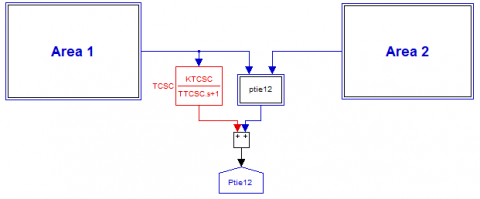Figure 1. MATLAB/SIMULINK model of two-area system

## 2.png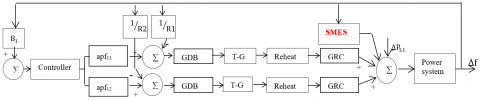Figure 2. Inside view of the first area

3. Flexible AC Transmission System Facts

FACTS is static equipment used for the AC transmission of electrical energy. It is meant to enhance controllability and increase power transfer capability . Figure 3 presents the different types of FACTS.

In this study, we are interested by TCSC unit.

## 3.pngFigure 3. Different generation FACTS controllers

3.1 Thyristor controlled series compensator

TCSC is an effective and economical means of solving problems of transient stability, dynamic stability, steady state stability and voltage stability in long transmission lines .

TCSC is connected in series with the tie line. Considering ∆f1(s) as the control signal to TCSC units, the transfer function of ∆Kc(s) may be shown in Eq. (1):

$\Delta K_{c}=\frac{K_{T C S C}}{1+T_{T C S C}} \Delta f_{1}(s)$                   (1)

where, KTCSC and TTCSC are the gain and tie constant of the TCSC controller respectively. Figure 4 presents the TCSC unit .

## 4.png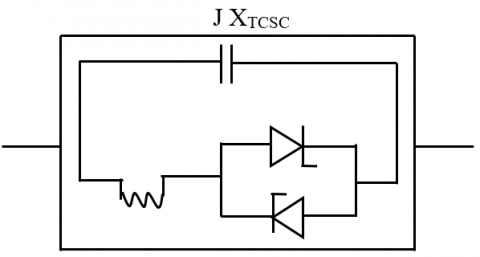Figure 4. TCSC unit

4. Superconducting Magnetic Energy Storage

SMES is an energy storage technology that store energy in the form of de electricity that is the source of a de magnetic field. The superconducting coil can be charged to a set point (which is less than the full charge) from the utility grid during normal operation of the grid [21-23].

A schematic diagram of SMES is shown in Figure 5.

## 5.png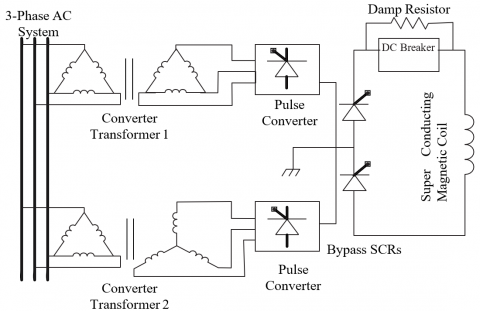Figure 5. SMES circuit diagram

5. Problem Formulated

5.1 Structure of PI-PD cascade controller

The regulator used in this study is a PI-PD cascade regulator. It is one of controller used to improve the better performance to the system.

Figure 6 described the block diagram of PI-PD cascade controller .

## 6.png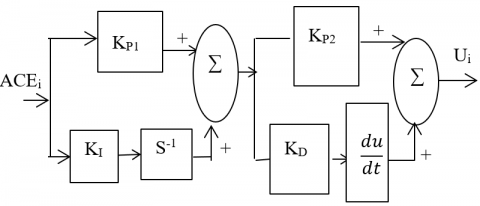Figure 6. Structure of PI-PD cascade controller

The error inputs to the controllers are given by:

$J=e_{i}=\sum_{i=1}^{n} A C E_{i}$              (2)

The main settings of the PI-PD controller have to be tuned using optimization algorithms by minimizing dynamical criteria. In our study, the optimization problem can be formulated as follows:

Minimize $\mathrm{J}\left(K_{P}, K_{I}, K_{D}\right)$

Subjected to $\left\{\begin{array}{l}K_{P, i}^{\min } \leq K_{P, i} \leq K_{P, i}^{\max } \\ K_{I, i}^{\min } \leq K_{I, i} \leq K_{I, i}^{\max } \\ K_{D, i}^{\min } \leq K_{D, i} \leq K_{D, i}^{\max }\end{array}\right.$

where, KP,i, KI,i, and KD,i are PI-PD controller gains of ith area. In this study, the parameter bounds limits are fixed between 0 and 10.

5.2 Firefly algorithm (FA)

Firefly is a swarm meta-heuristic algorithm and nature-inspired by the flashing behavior of fireflies. Figure 7 presents the flowchart of FA.

## 7.png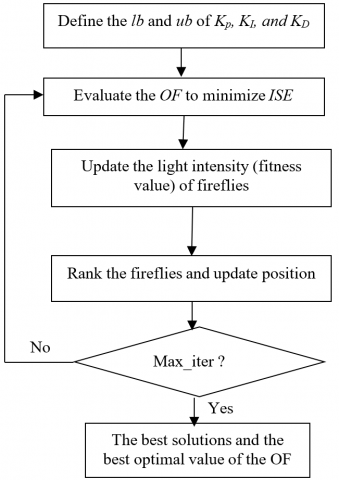Figure 7. Flowchart of FA

6. Simulation and Results

In order to check the efficiency of the both TCSC and SMES units, simulations were carried out on two-area two-unit reheat thermal-thermal systems as shown in Figure 1. The inside view of area one is presented in Figure 2. The suggested control scheme is considered in both areas. In all the study, we have considered 1% load variation in area 1. Then, the FA has been executed to obtain the optimal controllers settings by evaluating the ISE criterion. In this study, we have simulated three different cases as follows:

Case 1: in this first scenario, we have just considered the presence of the control scheme (PI-PD cascade controller).

Case 2: in the second scenario, the presences of TCSC unit in parallel with AC tie line and the control scheme.

Case 3: in the third scenario, we have considered the presence of all units such as TCSC, SMES and control scheme.

After running the optimisation procedure, the optimal obtained controllers’ parameters are tabulated in Table 1. The nominal system parameters are taken from  and offered in the Appendix. Table 2 presents the ISE based criterion in all three cases.

Table 1. Optimal PI-PD cascade controller settings using ISE based criterion

 KP1 KI KP2 KD Case 1 Area 1 3.0589 3.8672 4.9597 2.7826 Area 2 3.7712 4.2796 4.2466 2.9724 Case 2 Area 1 4.0175 2.4560 2.3172 0.4149 Area 2 1.0964 2.4463 1.8687 1.3612 Case 3 Area 1 2.1346 3.6352 2.5625 0.9133 Area 2 4.7444 7.7982 8.6262 4.9080

Table 2. Comparison of ISE criterion of Case 1, Case 2 and Case 3

 Case 1 Case 2 Case 3 ISE 0.0921 0.0553 0.0208

From Table 2, we remarked that the ISE of the third case has been decreased by 62.39 % and 77.41% comparatively to second and first cases respectively.

Figures 8-10 (a and b) present respectively the dynamical responses of ACEi, ∆fi and ∆Pmi with the consideration of GDB and GRC in all three cases. Figure 11 demonstrates the ∆Ptie12 in all cases.

It is clearly observed from Figures 8-10 that the better dynamic performance is obtained with the suggested third case compared to the second and first cases in terms of (S.T), (P.O), and (P.U).

The Settling Time (S.T), Peak Overshoot (P.O), and Peak Undershoot (P.U) of Figure 8a for all the cases are tabulated in Table 3.

## 8a.jpg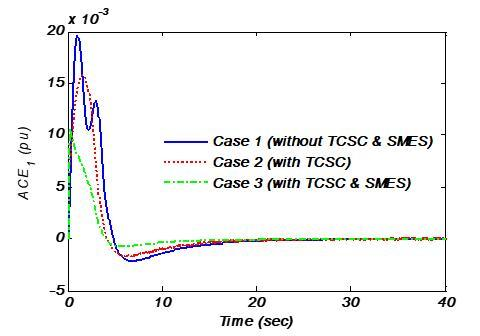(a)

## 8b.jpg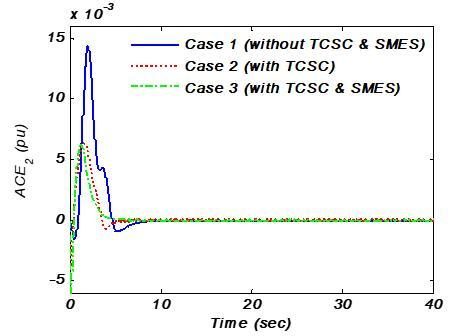(b)

Figure 8. Dynamical responses of (a): ∆f1 and (b): ∆f2 and for 1% load change in area one

## 9a.jpg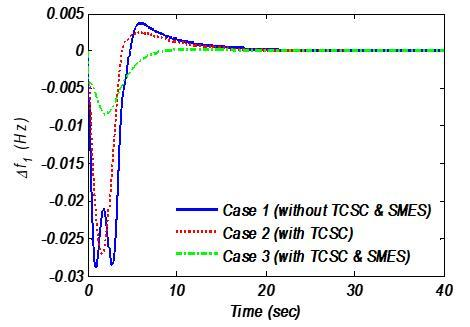(a)

## 9b.jpg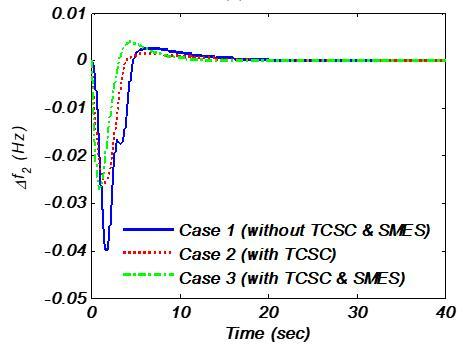(b)

Figure 9. Dynamical responses of (a): ACE1 and (b): ACE2 for 1% load change in area one

## 10a.jpg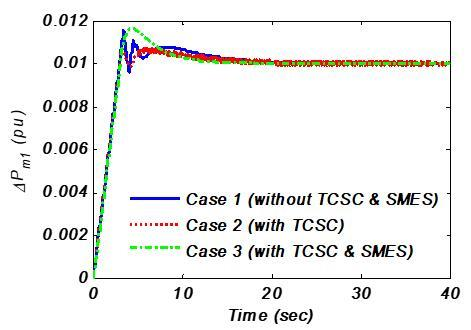(a)

## 10b.jpg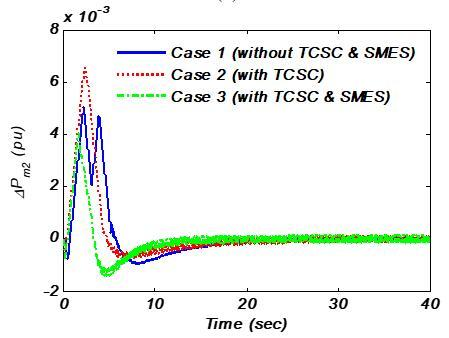(b)

Figure 10. Dynamical responses of (a): ∆Pm1 and (b): ∆Pm2 for 1% load change in area one

## 11.jpg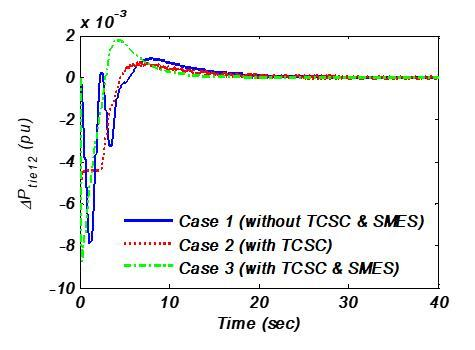Figure 11. Dynamical response of ∆Ptie12 for 1% load change in area one

Table 3. Settling Time (S.T), Peak Overshoot (P.O), and Peak Undershoot (P.U) of different frequency dynamical responses obtained with ISE

 P.O P.U S.T ACE 1 Case 1 0.0195 -2*10-3 14 Case 2 0.016 -1.7*10-3 12 Case 3 0.0105 -0.5*10-3 5

For example, the S.T of the third case has been decreased by 58%, 64% compared to the second and first cases respectively.

7. Conclusions

In this article, a FA optimised PI-PD cascade controller has been proposed for the AGC problem of two-area two-unit power systems with the consideration of TCSC and SMES units. The effects of some non-linear constraints such as GRC and GDB have been taken into account for more realistic study. Furthermore, three simulation scenarios have been considered as follows: scenario one: only the PI-PD cascade controller is considered, in the second scenario: we have added the TCSC unit in parallel with the control scheme, and finally, in the third scenario we have considered the presence of SMES unit in addition the previous scenario. From the obtained results, it is observed that significant improvements of dynamic responses are obtained with coordinated application of TCSC and SMES units from several views of point.

On the other hand, the following points can be recommended to complete this research:

(1) Implement and test the proposed system on a real power system;

(2) Analyse the AGC problem in a deregulated environment;

(3) Develop and design other control strategies that meet the AGC requirements and modern power system needs;

(4) Introduce other non-linear effects in the AGC model.

Appendix

Nominal parameters of the system investigated are:

B1=B2= 0.42249 p.u. MW/Hz; R1=R2=R3=R4=2.4 Hz/p.u.; TG1=TG2=TG3=TG4=0.08 s; TT1=TT2=TT3=TT4=0.3 s; KP=120 Hz/p.u.; TP=20s; KR1=KR2=10; TR1=TR2=10 s; T12=0.0866.

TCSC data:

KTCSC= 2.0; TTCSC=0.02 s.

Non-linear constraints:

GDB=±0.036Hz, and GRC=±10% per minute=0.0017 Pu.MW/min.

References

 Bevrani, H. (2014). Robust power system frequency control. Power Electronics and Power Systems University of Kurdistan Iran.

 Parsai, N., Thakur, A. (2015). PV curve approach for voltage stability analysis. International Journal of Scientific Research Engineering and Technology (IJSRET), 4: 373-377.

 Farmer, R.G. (2001). Power system dynamics and stability. The Electrical Power Engineering Handbook, Ed, L.L. Grigsby, Boca Raton: CRC Press LLC.

 Bevrani, H. (2004). Decentralized robust load-frequency control synthesis in restructured power systems. PhD Thesis, Department of Electrical Engineering, Graduate School of Engineering, Osaka University.

 Topno, P.N., Chanana, S. (2015). Tilt integral derivative control for two-area load frequency control problem. Proceedings of RAECS UIET Conference, Panjab University Chandigarh, Chandigarh, India. https://doi.org/10.1109/RAECS.2015.7453361

 Sahu, R.K., Panda, S., Biswal, A., Sekhar, G.T.C. (2016). Design and analysis of tilt integral derivative controller with filter for load frequency control of multi-area interconnected power systems. ISA Transactions, 61: 251- 264. https://doi.org/10.1016/j.isatra.2015.12.001

 Topno, P.N., Chanana, S. (2016). Application of tilt integral derivative control on two-area power system. Journal of Engineering Research, 4: 4-12. https://doi.org/10.7603/s40632-016-0012-4

 Delassi, A., Arif, S., Mokrani, L. (2016). A novel tilt integral derivative plus second derivative order for load frequency control problem in power system. 8th International Conference on Modelling, Identification and Control (ICMIC-2016), Medea and Algiers, Algeria, pp. 359-363. https://doi.org/10.1109/ICMIC.2016.7804137

 Shankar, R., Kumar, A., Raj, U., Chetterjee, K. (2018). Fruit fly algorithm based automatic generation control of multi-area interconnected power system with FACTS and AC/DC links in deregulated power environment. International Transactions on Electrical Energy Systems, 29(1): e2690. https://doi.org/10.1002/etep.2690

 Khamari, D., Sahu, R.K., Panda, S. (2019). Application of search group algorithm for automatic generation control of multi-area multi-source power systems. In E3S Web of Conferences, 87: 01005.

 Pathak, N., Verma, A., Bhatti, T.S., Nasiruddin, I. (2018). Modeling of HVDC tie links and their utilization in AGC/LFC operations of multiarea power systems. IEEE Transactions on Industrial Electronics, 66(3): 2185-2197. https://doi.org/10.1109/TIE.2018.2835387

 Abd-Elazim, S.M., Ali, E.S. (2018). Load frequency controller design of a two-area system composing of PV grid and thermal generator via firefly algorithm. Neural Computing and Applications, 30(2): 607-616. https://doi.org/10.1007/s00521- 016-2668-y

 Gorripotu, T.S., Sahu, R.K., Panda, S. (2015) Comparative performance analysis of classical controllers in LFC using FA technique. In Proc. of 2015 Int. Conf. on Elect., Electronics, Signals, Communication and Optimization (EESCO), Visakhapatnam, India, pp. 1-5.

 Delassi, A., Arif, S., Mokrani, L. (2015). Load frequency control problem in interconnected power systems using robust fractional PIλD controller. Ain Shams Engineering Journal, 9(1): 77-88. https://doi.org/10.1016/j.asej.2015.10.004

 Padhy, S., Panda, S., Mahapatra, S. (2017). A modified GWO technique based cascade PI-PD controller for AGC of power systems in presence of plug in electric vehicles. Engineering Science and Technology, an International Journal, 20(2): 427-442. https://doi.org/10.1016/j.jestch.2017.03.004

 Ullah, K., Basit, A., Ullah, Z., Aslam, S., Herodotou, H. (2021). Automatic generation control strategies in conventional and modern power systems: A comprehensive overview. Energies, 14: 2376. https://doi.org/10.3390/en14092376

 Singh, S.P., Prakash, T., Singh, V.P., Babu, M.G. (2017). Analytic hierarchy process based automatic generation control of multi-area interconnected power system using Jaya algorithm. Engineering Applications of Artificial Intelligence, 60: 35-44. https://doi.org/10.1016/j.engappai.2017.01.008

 Sahu, R.K., Panda, S., Padhan, S. (2015). A hybrid firefly algorithm and pattern search technique for automatic generation control of multi area power systems. International Journal of Electrical Power & Energy Systems, 64: 9-23. https://doi.org/10.1016/j.ijepes.2014.07.013

 Yousfi, B., Delassi, A., Arif, S. (2018). Synthesis of swarm based algorithms for the design of LFC in interconnected power system. CISTEM IEEE, pp. 1-6. https://doi.org/10.1109/CISTEM.2018.8613438

 Khatoon, N., Shaik, S. (2017). A survey on different types of flexible ac transmission systems (FACTS) controllers. International Journal of Engineering Development and Research, pp. 796-813.

 Padhan, S., Sahu, R.K., Panda, S. (2014). Automatic generation control with thyristor controlled series compensator including superconducting magnetic energy storage unit. Ain Shams Engineering Journal, 5(3): 759-774. https://doi.org/10.1016/j.asej.2014.03.011

 Buckles, W., Hassenzahl, W.V. (2000). Superconducting magnetic energy storage. IEEE Power Engineering Review, 20(5): 16-20. https://doi.org/10.1109/39.841345

 Tripathy, S.C., Balasubramanian, R., Nair, P.C. (1992). Adaptive automatic generation control with superconducting magnetic energy storage in power systems. IEEE Transactions on Energy Conversion, 7(3): 434-441. https://doi.org/10.1109/60.148563

 Pradhan, P.C., Sahu, R.K., Panda, S. (2016). Firefly algorithm optimized fuzzy PID controller for AGC of multi-area multi-source power systems with UPFC and SMES. Engineering Science and Technology, an International Journal, 19(1): 338-354. https://doi.org/10.1016/j.jestch.2015.08.007